# A New Intelligent Fault-Tolerant Control Scheme for Wind Energy Systems under Actuator Faults

A New Intelligent Fault-Tolerant Control Scheme for Wind Energy Systems under Actuator Faults
LISAC Laboratory, Department of Physics, Faculty of Sciences Dhar Mehraz, Sidi Mohamed Ben Abdellah University, BP 1796 Atlas Fez 30000, Morocco

Corresponding Author Email:
ayoub.elbakri@usmba.ac.ma
Page:
265-272
|
DOI:
https://doi.org/10.18280/ejee.220307
17 March 2020
|
Accepted:
26 May 2020
|
Published:
30 June 2020
| Citation

OPEN ACCESS

Abstract:

A reliable fault-tolerant control model for wind turbines (WTs) is a prominent task to meet the control objectives under the presence of the actuator faults. To this aim, the main focus of the present article is the design of a new fault-tolerant control scheme to deal with both additive and multiplicative faults. The design of the proposed method includes two extreme learning machine (ELM) blocks; an ELM-baseline controller to keep the desired wind turbine performances, and fault-tolerant ELM-block to eliminate any possible actuator faults effect. The design process of the ELM controller and the ELM fault estimator are formulated in a way that they only depend on WT input-output data, which allows a much faster and more precise fault tolerance. The effectiveness of the ELM fault-tolerant method is tested and compared to a sliding mode observer-based accommodation method using a 5-MW WT benchmark model. Simulation results validate the great performances of the proposed method.

Keywords:

wind turbines, fault-tolerant control (FTC), actuator fault, extreme learning machine, multiplicative faults, additive faults

1. Introduction

Wind energy is renewable, clean, and inexpensive alter- native energy. However, the amount of energy generated by wind turbines depends on the available wind speed. So, to perfectly benefit from the available wind energy, the wind turbine plant become larger and more sophisticated. Within the wind turbine systems, the servo pitch subsystem control is of paramount importance for mitigating the operational load and limiting the power capture under high wind speeds . Numerous pitch control strategies developed for wind turbines in the literature. These methods usually classified into two main categories; Individual pitch control methods (ICP) [2, 3], where each pitch position control controller designed separately. The collective pitch control methods (CPC) [4, 5], where the pitch positions of all blades controlled together using the same pitch angle reference. In the CPC framework, many strategies have been suggested including, the PID control , the gain-scheduling control method , the Linear Parameter-Varying control , and the Linear Quadratic Gaussian control method . However, the high-performance of the pitch control is still a considerable challenge due to the nonlinear properties of the wind turbine aerodynamics and the strong coupling of the wind turbine subsystems. Besides, in real operation, pitch systems may have actuator faults that can cause the system to shut down or even to be broken if proper measures not held . In this matter, the fault-tolerant control method is a potent technique that elaborated over the past decades to tolerate faults and maintain an acceptable performance level [11, 12]. According to the proposed FTC methods, two main categories can be distinguished when dealing with faults. Passive FTC methods where a robust controller designed so that the system can operate during fault-free and faulty cases and active fault-tolerant techniques where the controller is adjusted based on the available information on the fault function. When browsing the passive FTC methods [13-15], one can notice that the control low does not require to be changed when the fault appears in the system. In other terms, this means that the design steps of the controller need to calculate the parameters for the most severe case that the system can handle, which is disadvantageous because it increases the complexity of the controller. Moreover, the worst fault scenario can be different for each wind turbine on account of varying factors as for the external environment and the WT technology been used.

On the other hand, the active FTC method has become a new focus [16-18]. The fact that the controller can benefit from the fault information obtained based on the changes of the system state variables when aroused by faults has the advantage of fast fault compensation, which can optimally recover the nominal operation status. However, the system state variables not always available, thus a state estimator is to be included in the design process, which degrade the control performances due to the estimation error, the neglected dynamics, and the reduced model used to describe the wind turbine system. Moreover, as in the study , the designed controller is usually based on a linearized WT model around a fixed operating point, which may not provide identical performance when the operating point or the WT parameters deviate significantly. Furthermore, the significant attention of the proposed studies on the FTC of WT concentrates on additive faults, thus only a few efforts made on multiplicative actuator faults.

The main objectives of this paper are to examine the following practical requirements: (i) design a robust ELM- controller with fast and reduced fluctuation in the full load region. (ii) estimate the changes in the control performances caused by actuator faults (iii) apply FTC to recover the nominal pitch performance. In specific, two ELM models built for this reason. An ELM-baseline controller formulated to depends only on the available WT outputs and a fast ELM fault estimator where the representation form for multiplicative faults written so that those faults estimated from the input-output information.

The rest of this work is as follows. Section 2 presents the WT system with concerned actuator faults, along with a de- tailed introduction to the ELM method. Section 3 details the processes of considered FTC control method. The efficiency of the ELM-based FTC method investigated in Section 4. Finally, the conclusion provided in Section 5.

2. Problem Formulation

2.1 Wind turbine modeling and faults

As detailed in Figure 1, the entire wind turbine system is the interconnection between several subsystems: aerodynamics, the pitch subsystem, the generator unit, and the mechanical subsystem, which comprises two blocks, i.e., the drive-train dynamics, and the support structure .

## 1.png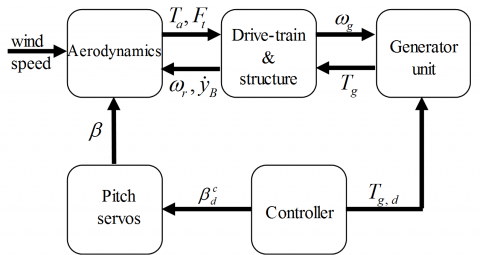Figure 1. Connection between the wind turbine subsystems

In this work, we only focus on faults affecting the pitch actuator. Therefore, only the pitch control system and the dynamics used to introduce the ELM controller described in this section. However, in the simulation section, the complete FAST wind turbine model is employed to test the proposed ELM-FTC method. Moreover, since the wind turbine has tree individual pitch actuators, it is assumed that these actuators have the same dynamic structure and the same control input. The equations of motion describing the dynamics of each pitch actuator and the rotational speeds are defined as follows.

$\dot{\beta }={{\alpha }_{\beta }}\left( -\beta +\beta _{d}^{c} \right)$           (1)

${{J}_{r}}{{\dot{\omega }}_{r}}+{{b}_{s}}\left( {{\omega }_{r}}-{{\omega }_{g}} \right)+{{d}_{s}}{{\phi }_{s}}={{T}_{a}}$             (2)

${{J}_{g}}{{\dot{\omega }}_{g}}-{{b}_{s}}\left( {{\omega }_{r}}-{{\omega }_{g}} \right)-{{d}_{s}}{{\phi }_{s}}=-{{T}_{g}}$            (3)

where, $\beta$, $\beta_{d}^{c}$ are the actual pitch and the desired control command, αβ is the constant related to the pitch system. ωr, ωgsymbolize the angular velocities of the rotor and the generator, Jr, Jgdenote the corresponding inertias. As for ϕs, ds and bs they indicate the torsional angle, stiffness and damping coefficient respectively, and Tg indicates the electrical torque. The aerodynamic torque Ta  acting on the blades is modeled by

${{T}_{a}}=\frac{1}{2\lambda }\rho \pi {{R}^{3}}{{C}_{p}}(\lambda ,\beta ){{v}^{2}}$         (4)

where, Cp is the turbine power-coefficient, R is the radius of the blades, $\rho$ express the density of the air, and $\lambda=\omega_{r} R / v$ is called the speed ratio.

As each of the tree pitch systems can be subject to faults triggered as a change in the actuator dynamics (as an offset in the value of αβ), the faulty actuator is modeled in the following form:

$\dot{\beta }=-{{\alpha }_{\beta 0}}\beta +{{\alpha }_{\beta 0}}\beta _{d}^{c}+{{f}_{\beta }}$        (5)

where, $f_{\beta}=\left(\alpha_{\beta f}-\alpha_{\beta 0}\right)\left(\beta_{d}^{c}-\beta\right)$ is the fault function in which αβ0 is the nominal value of αβ, and αβ,f is the value when fault appear in the actuator. To respect the physical constraints of actuators as a rate limiter is implemented to the controller as illustrated in Figure 2.

## 2.png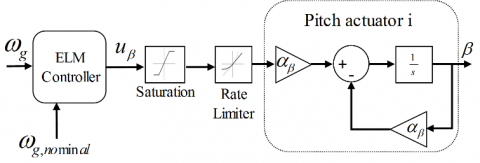Figure 2. The pitch actuator model

2.2 The ELM algorithm

The extreme learning machine algorithm is a kind of neural networks with only one hidden layer that is developed to estimate complex nonlinear dynamics from the available input samples [21-23]. The central idea of the ELM algorithm is that the input weights vector that connects the input nodes with the hidden layer are randomly attributed if the activation function allocated to the hidden layer is chosen infinitely differentiable. Hence, when the weight vector is fixed, the ELM can be seen as a linear system, and the output weight vector can be computed using the generalized inverse operation. From mathematical perspective, for L arbitrary different samples (xi, ti) where $x_{i} \in \mathfrak{R}^{p}$ and $t_{i} \in \mathfrak{R}^{q}$  the ELM network with M hidden nodes and activation function w(x) can be expressed as

$\sum\limits_{i=1}^{M}{{{\theta }_{i}}w({{\mathbf{x}}_{j}})=}\sum\limits_{i=1}^{M}{{{\theta }_{i}}w({{\mathbf{a}}_{i}}{{\mathbf{x}}_{j}}+{{b}_{i}})={{\mathbf{y}}_{j}}}\,\,\,\,\,\,\,\,\,\,\,\,\,j=1,\ldots ,L\,\,\,$                  (6)

where, $\mathbf{a}_{i} \in \mathfrak{R}^{p}$ is the input weight vector, $\theta_{i}$ is the output weight vector and bi is the bias. Given the training data (xi, ti) if the ELM output is equal to desired target then there exist a weight vectors θi, ai and bi satisfies the following statement:

$\sum\limits_{i=1}^{M}{{{\theta }_{i}}{{w}_{i}}({{\mathbf{a}}_{i}}{{\mathbf{x}}_{j}}+{{b}_{j}})={{\mathbf{t}}_{j}}}$         (7)

which can be rewritten in the matrix form as

$\mathbf{\theta }\,\mathbf{W}=\mathbf{T}$               (8)

To extract the output weight, a special solution of the system (8) is calculated by

$\mathbf{\theta }={{\mathbf{W}}^{\dagger }}\mathbf{T}$      (9)

where, ${{\mathbf{W}}^{\dagger }}$ and T denote the generalized inverse of W, and the target vector given by

$\mathbf{W}=\left( \begin{matrix} w({{a}_{1}}{{x}_{1}}+{{b}_{1}}) & \cdots & w({{a}_{M}}{{x}_{1}}+{{b}_{M}}) \\ \vdots & \cdots & \vdots \\ w({{a}_{1}}{{x}_{L}}+{{b}_{1}}) & \cdots & w({{a}_{M}}{{x}_{L}}+{{b}_{M}}) \\\end{matrix} \right)$, $\mathbf{\theta }=\left( \begin{matrix} \theta _{1}^{T} \\ \vdots \\ \theta _{M}^{T} \\\end{matrix} \right)$ and $\mathbf{T}=\left( \begin{matrix} t_{1}^{T} \\ \vdots \\ t_{L}^{T} \\\end{matrix} \right)$.

Lemma 1 : Given a scalar $\gamma>0$ and any differentiable activation function $w:R\to R$, there exist M<L such that for L arbitrary different samples (xi,ti), for any random wight input vector (ai,bi) chosen according to any continuous probability the following statement holds: $\left\| \mathbf{W\theta }-\mathbf{T} \right\|<\gamma$.

3. Elm-Based FTC Method

In this section, the ELM baseline controller is proposed first to maintain constant power generation under the high wind speed. Then, the FTC strategy is developed for each pitch actuator to recover the nominal dynamic behaviour when fault affects one of the actuators (see Figure 3).

3.1 ELM-baseline controller

The baseline controller for the pitch system only activated in the high operating zones of the wind speed. The concept is that the controller needs to acts on the system to change the pitch angle in order to limit the aerodynamic force acting on the blades to keep the generator rotational speed at the desired value, which thus reduces the load fatigue and prevent severe damages on the system. In respect, let assume that the wind turbine operates at the desired generator speed $\omega_{g, n o m}$, then, the torque is set as $T_{g}=P_{g, d} / \omega_{g, \text {nom}}$ and the governing equation modeling the rotational speed of the generator dynamics is given by:

${{\dot{\omega }}_{g}}=\frac{1}{{{J}_{r}}+{{J}_{g}}}(\frac{1}{2{{\omega }_{g}}}\rho \pi {{R}^{2}}{{C}_{p}}(\lambda ,\beta ){{v}^{3}}-\frac{{{P}_{g,d}}}{{{\omega }_{g,nom}}})$            (10)

where, Pg,d is the wind turbine nominal power. As can be observed from (10) the rotational speed can be simply rewritten as

${{\dot{\omega }}_{g}}=f({{\omega }_{g}},\beta ,v)$              (11)

As we can see also from (10), the pitch input is an implicit function in this equation. Let consider the following change:

$\eta ={{\dot{\omega }}_{g}}=f({{\omega }_{g}},{{\beta }^{*}},v)$              (12)

where, $u_{\beta }^{*}={{\beta }^{*}}={{f}^{*}}({{\omega }_{g,nom}},\eta ,v)$ is the ideal control function. Then, using the approximation theorem of the ELM algorithm, the controller $u_{\beta }^{*}$ is described as

$u_{\beta }^{*}=\mathbf{W}(v,{{\omega }_{g}},\eta ){{\theta }^{*}}+{{\varepsilon }_{\beta }}=\mathbf{W}(\mathbf{x}){{\theta }^{*}}+{{\varepsilon }_{\beta }}$          (13)

where, $\theta^{*}$ is the weight vectors of the optimal and the ELM controller, W(x) is the processed ELM hidden matrix, and $\varepsilon_{\beta}$ is a small bounded approximation error ($\left| {{\varepsilon }_{\beta }} \right|<{{\bar{\varepsilon }}_{\beta }}$). Therefore, ELM network depicted in Figure 4 can be set to approximate the optimal pitch command $u_{\beta }^{*}$ as follows:

${{u}_{\beta }}=\mathbf{W}(\mathbf{x})\theta$            (14)

3.1.1 Closed loop stability analysis

Let define the tracking error $e=\omega_{g}-\omega_{g, n o m}$. Hence, the dynamic of the error is obtained as:

$\dot{e}={{\dot{\omega }}_{g}}-{{\dot{\omega }}_{g,nom}}=f({{\omega }_{g}},{{u}_{\beta }},v)-{{\dot{\omega }}_{g,nom}}$         (15)

Let define the pseudo control $\eta$ as

$\eta ={{\dot{\omega }}_{g,nom}}-{{k}_{1}}e$          (16)

Given that $\eta$ is not a function of the input uβ but it only a state-dependent function it follows that in a neighborhood of each ωg, uβ, v, it results that

$\eta -f({{\omega }_{g}},{{f}^{*}}(v,{{\omega }_{g}},\eta ),v)=0$          (17)

## 3.pngFigure 3. The ELM-based FTC schemes

## 4.png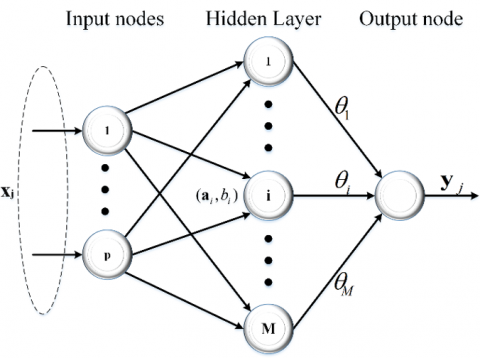Figure 4. The ELM baseline controller

Hence, using the mean value theorem, the dynamics of the error rewritten as

\begin{align} & \dot{e}=f({{\omega }_{g}},{{\beta }^{*}},v)+({{u}_{\beta }}-{{\beta }^{*}}){{\xi }_{\beta }}-{{{\dot{\omega }}}_{g,nom}} \\ & \,\,\,\,=\,\eta +\,({{u}_{\beta }}-{{\beta }^{*}}){{\xi }_{\beta }}-{{{\dot{\omega }}}_{g,nom}} \\ \end{align}          (18)

where, ${{\xi }_{\beta }}=\frac{\partial f({{\omega }_{g}},\bar{\beta },v)}{\partial \beta }$ with $\bar{\beta }=\lambda \beta +(1-\lambda )\beta$ and $\lambda \in (0,1)$. Let consider the following assumption

\begin{align} & -{{{\underset{\scriptscriptstyle-}{f}}}_{1}}\le \frac{\partial f({{\omega }_{g}},\bar{\beta },v)}{\partial \beta }\le -{{{\bar{f}}}_{2}}<0 \\ & \,\,\,\,\,\,\,\,\,\,\le N\left| \frac{d}{dt}(\frac{\partial \eta }{\partial \beta }) \right| \\ \end{align}                  (19)

where, ${{\underset{\scriptscriptstyle-}{f}}_{1}},{{\bar{f}}_{2}}$ and N are positive constants. Then, the following Lyapunov function is considered:

${{V}_{1}}=\,\frac{{{e}^{2}}}{2{{\xi }_{\beta }}}\,+\frac{1}{2\mu }{{\tilde{\theta }}^{T}}\tilde{\theta }$           (20)

where, $\tilde{\theta }=\theta -{{\theta }^{*}}$ is the weight error and $\mu$ is a positive scalar. Based on (16) and (18) the time derivative of V1 yields:

${{\dot{V}}_{1}}=\left( \frac{{{k}_{1}}}{{{\xi }_{\beta }}}+\frac{1}{2\xi _{\beta }^{2}} \right){{e}^{2}}+{{\tilde{\theta }}^{T}}\left( -{{\mathbf{W}}^{T}}(\mathbf{x})e+\frac{1}{\mu }\dot{\theta } \right)+e{{\varepsilon }_{\beta }}$           (21)

To ensure the robustness of the output weight, the e-modification is considered to update the output weights

$\dot{\theta }=\mu ({{\mathbf{W}}^{T}}(\mathbf{x})e-\tau \left| e \right|\theta )$                 (22)

where, $\tau>0$ and μ is the learning rate, using assumption (19) we obtain:

${{\dot{V}}_{1}}\le \left( -\frac{{{k}_{1}}}{{{{\underset{\scriptscriptstyle-}{f}}}_{1}}}+\frac{N}{2\bar{f}_{2}^{2}} \right){{e}^{2}}\,+\left| e \right|{{\bar{\varepsilon }}_{\beta }}+{{\tilde{\theta }}^{T}}\left( -{{\mathbf{W}}^{T}}(\mathbf{x})e+\frac{1}{\mu }\dot{\theta } \right)$ \begin{align} & {{{\dot{V}}}_{1}}\le \left( -\frac{{{k}_{1}}}{{{{\underset{\scriptscriptstyle-}{f}}}_{1}}}+\frac{N}{2\bar{f}_{2}^{2}} \right){{e}^{2}}\,+\left| e \right|{{{\bar{\varepsilon }}}_{\beta }}-\tau \left| e \right|{{{\tilde{\theta }}}^{T}}\theta \\ & \,\,\,\,\,\,\le \left( -K\left| e \right|+{{{\bar{\varepsilon }}}_{\beta }}-\tau {{{\tilde{\theta }}}^{T}}\theta \right)\left| e \right| \\\end{align}          (23)

which is equivalent to:

${{\dot{V}}_{1}}\le \left( -K\left| e \right|+{{{\bar{\varepsilon }}}_{\beta }}+\tau {{{\tilde{\theta }}}^{T}}{{\theta }^{*}}-\tau {{{\tilde{\theta }}}^{T}}\tilde{\theta } \right)\left| e \right|$

Hence, if $\left| e \right|\ge {({{{\bar{\varepsilon }}}_{\beta }}}/{K}\;)+{\tau {{\theta }^{2}}}/{4K}\;$ or $\left\| \theta \right\|\ge {\theta }/{2}\;+\sqrt{{{{\theta }^{2}}}/{2}\;+{{{{\bar{\varepsilon }}}_{\beta }}}/{\tau }\;}$ implies that ${{\dot{V}}_{1}}\le 0$, and that the closed loop system is uniformly bounded.

3.2 FE and FTC design

As outlined in Figure 3 beside the ELM baseline controller, the ELM-FTC scheme includes a fault estimation block along with a fault corrector to compensate the fault effects.

In regards to the fault estimator, as we can conclude from (5), the nonlinear actuator fault function fβ depends on the measured pitch output β=βm and the baseline controller uβ. Thus, the problem of estimating fβ can be also formulated using the ELM theorem as follows:

${{f}_{\beta }}=\,{{\mathbf{W}}_{f}}({{u}_{\beta }},{{\beta }_{m}})\theta _{f}^{*}+{{e}_{f}}={{\mathbf{W}}_{f}}(\mathbf{x})\theta _{f}^{*}+{{e}_{f}}$                 (24)

where, $\theta _{f}^{*}$ is the ideal output weight vector of the fault, and ef is the approximation error. According to lemma 1 the fault fβ can be also estimated using The ELM as follows:

${{\hat{f}}_{\beta }}={{\mathbf{W}}_{f}}(\mathbf{x}){{\mathbf{\theta }}_{f}}$               (25)

which can be seen as to find the weight vector $\boldsymbol{\theta}_{f}$ so that the tracking error ${{e}_{f}}={{f}_{\beta }}-{{\hat{f}}_{\beta }}$ converge to a small set ${{\bar{e}}_{f}}$ with $\left| {{e}_{f}} \right|<{{\bar{e}}_{f}}$. Wf is the fault estimator hidden matrix.

Finally, to achieve the fault tolerance, given that actuator faults modeled as additive faults, a simple fault compensation method is adopted as follows:

${{u}_{corr}}={{u}_{\beta }}-\frac{1}{{{\alpha }_{\beta ,0}}}{{\hat{f}}_{\beta }}$         (26)

such that when applying ucorr, the actual system becomes

$\dot{\beta }=-{{\alpha }_{\beta ,0}}\beta +{{\alpha }_{\beta ,0}}({{u}_{\beta }}-\frac{1}{{{\alpha }_{\beta ,0}}}{{\hat{f}}_{\beta }})+{{f}_{\beta }}$              (27)

which correspond to the original fault free case when ${{e}_{f}}\to 0$.

3.2.1 Closed loop stability analysis

Let consider the Lyapunov function given as

$V={{V}_{1}}+\frac{1}{2}e_{f}^{2}$            (28)

According to lemma 1 the fault estimation error ef bounded ($\left| {{e}_{f}} \right|<{{\bar{\varepsilon }}_{f}}$) let assume $\left| {{{\dot{e}}}_{\beta }} \right|<{{\rho }_{f}}$. Using (23), the time derivative of V becomes:

$\dot{V}\le \left( -K\left| e \right|+{{{\bar{\varepsilon }}}_{\beta }}-\tau {{{\tilde{\theta }}}^{T}}\theta \right)\left| e \right|+{{\dot{e}}_{f}}{{e}_{f}}$             (29)

Let $\delta ={{{{\bar{\varepsilon }}}_{f}}{{\rho }_{f}}}/{K}\;$ then if $\left| e \right|\ge {({{{\bar{\varepsilon }}}_{\beta }}}/{K}\;)+{\tau {{\theta }^{2}}}/{4K}\;+\delta$ or $\left\| \theta \right\|\ge {\theta }/{2}\;+\sqrt{{{{\theta }^{2}}}/{2}\;+{{{{\bar{\varepsilon }}}_{\beta }}}/{\tau }\;}$ then the Lyapunov function $\dot{V}\le 0$ and the closed-loop system is uniformly bounded.

4. Simulation Results

To illustrate the effectiveness of the proposed ELM-FTC scheme, we consider a 5 MW wind turbine whose parameters are given in the appendix.

## 5.png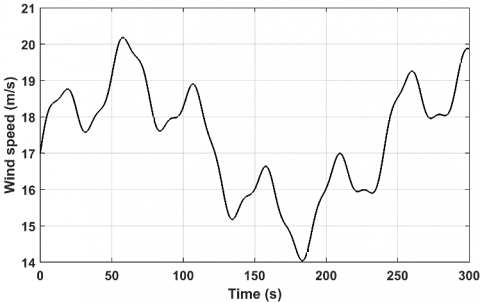Figure 5. The wind speed profile

During simulation we assume that the WT operate with a typical wind speed in the range between v= [11.2 25] m/s as depicted in Figure 5.

The control objective is to limit the generated power at the rated value by considering the physical system constraints, nonlinearities, and the presence of actuator faults. To this end, simulations performed for two cases. (i) the nominal case by implementing the proposed ELM-baseline controller without any actuator fault occurrence. (ii) the faulty case under the effect of actuator faults on the pitch actuator.

4.1 Case 1: Fault free

In this case, the ELM-baseline controller is tested using the wind sequence in Figure 5. The ELM controller parameters are set as follows; the activation function w(x) is a sigmoid function,

$w(x)=\frac{1}{1+{{e}^{-(ax+b)}}}$          (30)

while the number of hidden nodes is chosen so that the training error is close to zero, as indicated in Figure 6.

Next, to investigate the controller, robustness Figure 7 illustrates the ELM controller with different parameters setup where the input weight vector is chosen randomly in the interval [-1 1].

## 6.png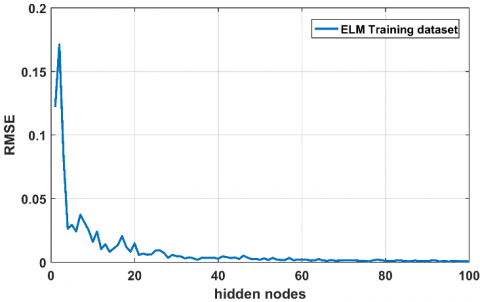Figure 6. Baseline controller training error

## 7.png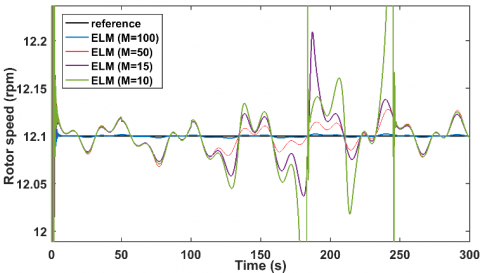Figure 7. ELM controller with different parameters

## 8.pngFigure 8. The rotor speed output

## 9.png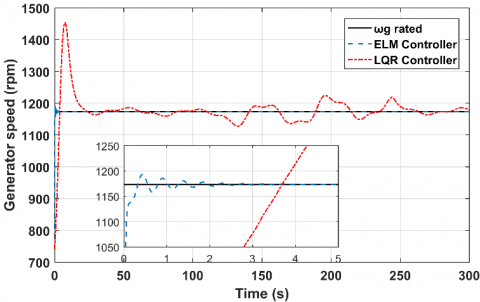Figure 9. The generator speed output

## 10.png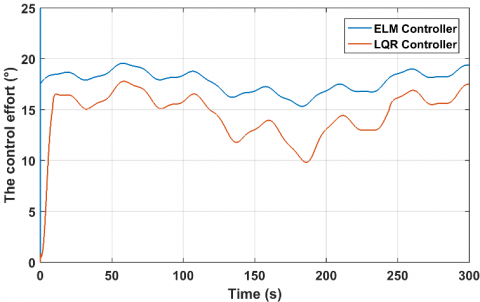Figure 10. The control effort

From Figure 7, we can observe that the ELM controller can achieve acceptable tracking performance with a small number of neurons in the hidden layer.

In the following, to further asses the performance of the method, the LQR control method  is employed as a comparative study. Figures 8-10. shows the angular velocities of the rotor, generator and the control effort made by both controllers.

From these plots it can be seen that the ELM controller produces robust responses compared to the LQR controller which clearly manifest in term of the reduced fluctuation generated by the ELM controller compared to the LQR controller, and in the fast convergence to the desired value.

4.2 Case 2: Faulty actuator

Next, to check the fault tolerance performance of the ELM method, two actuator faults have been considered for the pitch actuators. A multiplicative fault represented as a 20% loss in the capacity of the actuator between the time 100s and 130s $\left(u_{f}(t)=0.8 u_{\beta}(t)\right)$. And an offset actuator fault represented as an additive fault of fa2=0.5rad/s at the time t=50s.

In a similar way to the baseline ELM controller, the design parameters of the ELM estimator are set using 100 hidden nodes and processed using the sigmoid function. In Figures 11-12, the performance of fault estimation is compared with the sliding mode observer  using the ELM baseline controller.

## 11.png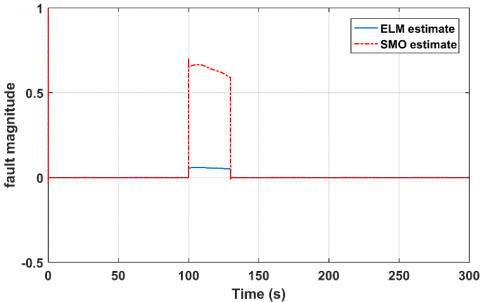Figure 11. Estimation of the multiplicative actuator fault fa1

## 12.png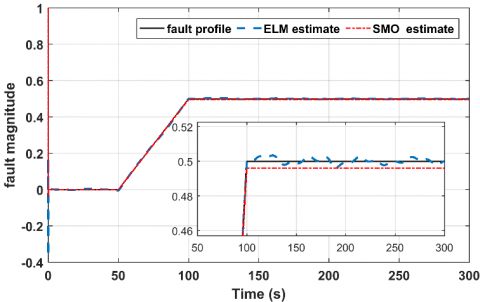Figure 12. Estimation of the offset actuator fault fa2

## 13.png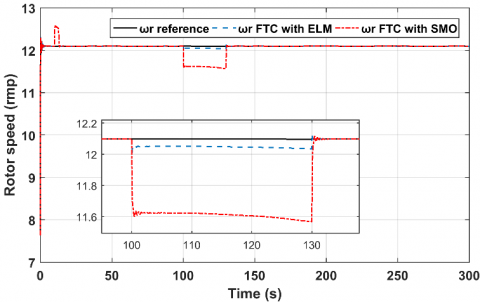Figure 13. Tracking of reference rotor speed with FTC in actuator fault fa1

## 14.png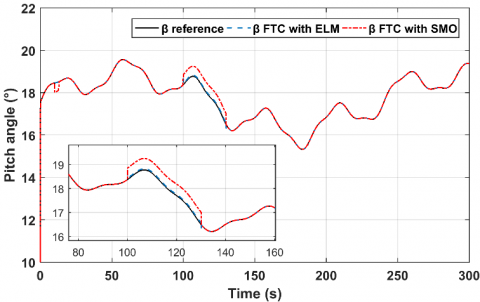Figure 14. Tracking of reference pitch position with FTC in actuator fault fa1

As shown in Figures 11 and 12, it can be seen that both the ELM estimator and the sliding mode observer produces accurate estimates of the actuator fault offset. However, for the multiplicative fault, the ELM method outperforms the SMO technique in terms of accuracy, which manifests when applying the FTC to eliminate the fault effect. Figures 13-16 shows the actual and the faulty rotor speed and the pitch position outputs when applying the tolerant control low (26) based on the estimate provided by both methods.

From the plots 13-16, it can be seen that the FTC based on the SMO does not converge to the desired quantities in the case of the fault fa2, and this is due to the poor estimation accuracy of the SMO when facing nonlinear distribution fault function as in fa1. On the other hand, when using the ELM-FTC scheme, the wind turbine outputs converge to the nominal values with excellent performance.

## 15.png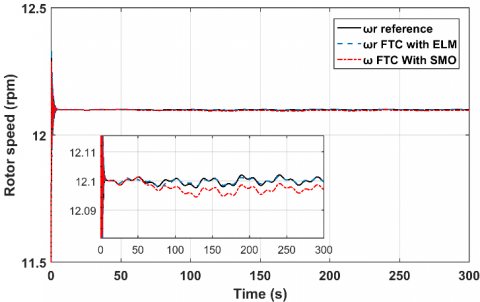Figure 15. Tracking of reference rotor speed with FTC in actuator fault fa2

## 16.png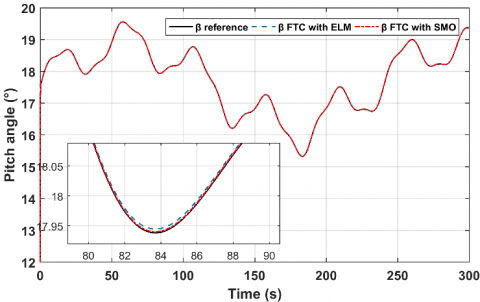Figure 16. Tracking of reference pitch position with FTC in actuator fault fa2

5. Conclusions

In this work, an ELM-based FTC method has been designed to achieve the desired control performance in the high wind speed operating zone and to eliminate any possible actuator fault in the pitch system. The method included two ELM models, one to provide the optimal control command in the fault-free case, and the second to approximate the actuator fault affecting the WT system. Using the information of the ELM estimation model, an active fault-tolerant compensator is presented and tested by numerical simulation. The performed simulation demonstrates that the ELM-FTC can recover the standard actuation, guarantee the WT stability, and ensure better control performance. For future research, it is essential to extend the method developed to manage sensor faults and tested it in several power resources.

Appendix

The wind turbine nominal parameters

${{P}_{g,d}}=5\,MW,$$\rho =1.225\,Kg/{{m}^{3}}$${{J}_{r}}=38759227\,Kg{{m}^{2}}$,${{J}_{g}}=5025347\,Kg{{m}^{2}}$, $R=63\,m$, ${{\omega }_{g,nom}}=1173.7\,rpm$, ${{b}_{s}}=867637000\,Nm$, the gear box ratio ${{n}_{g}}=97$,${{d}_{s}}=6215000\,Nm\,s$, $\tau =\frac{1}{{{\alpha }_{\beta 0}}}=0.1\,s$,$\beta \in {{\left[ \begin{matrix} 0 & 90 \\\end{matrix} \right]}^{0}}$.

References

 Odgaard, P.F., Stoustrup, J., Kinnaert, M. (2013). Fault-tolerant control of wind turbines: A benchmark model. IEEE Transactions on Control Systems Technology, 21(4): 1168-1182. http://dx.doi.org/10.1109/TCST.2013.2259235

 Zhang, D., Cross, P., Ma, X., Li, W. (2013). Improved control of individual blade pitch for wind turbines. Sensors and Actuators A: Physical, 198: 8-14. http://dx.doi.org/10.1016/j.sna.2013.04.020

 Namik, H., Stol, K. (2011). Performance analysis of individual blade pitch control of offshore wind turbines on two floating platforms. Mechatronics, 21(4): 691-703. https://doi.org/10.1016/j.mechatronics.2010.12.003

 Lasheen A., Elnaggar M., Haitham Y. (2019). Adaptive control design and implementation for collective pitch in wind energy conversion systems. ISA Transactions, 102: 251-263. https://doi.org/10.1016/j.isatra.2019.11.019

 Oh, K.Y., Park, J.Y., Lee, J.S., Lee, J. (2015). Implementation of a torque and a collective pitch controller in a wind turbine simulator to characterize the dynamics at three control regions. Renewable Energy, 79: 150-160. https://doi.org/10.1016/j.renene.2014.10.002

 Gambier, A., Nazaruddin, Y. (2018). Collective pitch control with active tower damping of a wind turbine by using a nonlinear PID approach. IFAC-PapersOnLine, 51(4): 238-243. https://doi.org/10.1016/j.ifacol.2018.06.072

 Leith, D.J., Leithead, W.E. (2000). Survey of gain scheduling analysis and design. International Journal of Control, 73(11): 1001-1025. https://doi.org/10.1080/002071700411304

 Inthamoussou, F.A., Battista, H.D., Mantz, R.J. (2016). LPV-based active power control of wind turbines covering the complete wind speed range. Renewable Energy, 99: 996-1007. http://dx.doi.org/10.1016/j.renene.2016.07.064

 Georg, S., Schulte, H. (2013). Actuator fault diagnosis and fault-tolerant control of wind turbines using a Takagi-Sugeno sliding mode observer. 2013 Conference on Control and Fault-Tolerant Systems (SysTol), pp. 516-522. https://doi.org/10.1109/SysTol.2013.6693872

 Hameed, Z., Hong, Y., Cho, Y., Ahn, S., Song, C. (2009). Condition monitoring and fault detection of wind turbines and related algorithms: A review. Renewable and Sustainable Energy Reviews, 13(1): 1-39. https://doi.org/10.1016/j.rser.2007.05.008

 Odgaard, P.F., Stoustrup, J. (2015). A benchmark evaluation of fault tolerant wind turbine control concepts. IEEE Transactions on Control Systems Technology, 23(3): 1221-1228. http://dx.doi.org/10.1109/TCST.2014.2361291

 Yu, X., Jiang, J. (2015). A survey of fault-tolerant controllers based on safety-related issues. Annual Reviews in Control, 39: 46-57. https://doi.org/10.1016/j.arcontrol.2015.03.004

 Benosman, M., Lum, K. (2010). Passive actuators’ fault-tolerant control for affine nonlinear systems. IEEE Transactions on Control Systems Technology, 18(1): 152-163. http://dx.doi.org/10.1109/TCST.2008.2009641

 Tabatabaeipour, S., Izadi-Zamanabadi, R., Bak, T., Ravn, A. (2012). Passive fault-tolerant control of discrete time piecewise affine systems against actuator faults. International Journal of Systems Science, 43: 1985-1997. https://doi.org/10.1080/00207721.2012.670289

 Badihi, H., Zhang, Y. (2017). Passive fault-tolerant cooperative control in an offshore wind farm. Energy Procedia 105. 8th International Conference on Applied Energy, Beijing, pp. 2959-2964. https://doi.org/10.1016/j.egypro.2017.03.697

 Simani, S., Castaldi, P. (2014). Active actuator fault-tolerant control of a wind turbine benchmark model. International Journal of Robust and Nonlinear Control, 24: 1283-1303. http://dx.doi.org/10.1002/rnc.2993

 Shaker, M.S., Patton, R.J. (2014). Active sensor fault tolerant output feedback tracking control for wind turbine systems via T-S model. Engineering Applications of Artificial Intelligence, 34: 1-12. https://doi.org/10.1016/j.engappai.2014.04.005

 Li, S., Wang, H., Aitouche, A., Christov, N. (2018). Active fault tolerant control of wind turbine systems based on DFIG with actuator fault and disturbance using Takagi–Sugeno fuzzy model. Journal of the Franklin Institute, 355: 8194-8212. https://doi.org/10.1016/j.jfranklin.2018.08.021

 Azizi, A., Nourisola, H., Shoja-Majidabad, S. (2019). Fault tolerant control of wind turbines with an adaptive output feedback sliding mode controller. Renewable Energy, 135: 55-65. https://doi.org/10.1016/j.renene.2018.11.106

 Georg, S., Schulte, H., Aschemann, H. (2012). Control oriented modelling of wind turbines using a Takagi-Sugeno model structure. 2012 IEEE International Conference on Fuzzy Systems, pp. 1-8. https://dx.doi.org/10.1109/FUZZ-IEEE.2012.6251302

 Bakri, A.E., Koumir, M., Boumhidi, I. (2019). Extreme learning machine-based non-linear observer for fault detection and isolation of wind turbine. Australian Journal of Electrical and Electronics Engineering, 16(1): 12-20. http://dx.doi.org/10.1080/1448837X.2019.1578044

 Huang, G.B., Zhu, Q.Y., Siew, C.K. (2006). Extreme learning machine: Theory and applications. Neurocomputing, 70: 489-501. https://doi.org/10.1016/j.neucom.2005.12.126

 Koumir, M., EL Bakri, A., Boumhidi, I. (2017). Integral sliding mode control based on extreme learning machine for a wind turbine. Control and Intelligent Systems, 45(3). http://dx.doi.org/10.2316/Journal.201.2017.3.201-2752